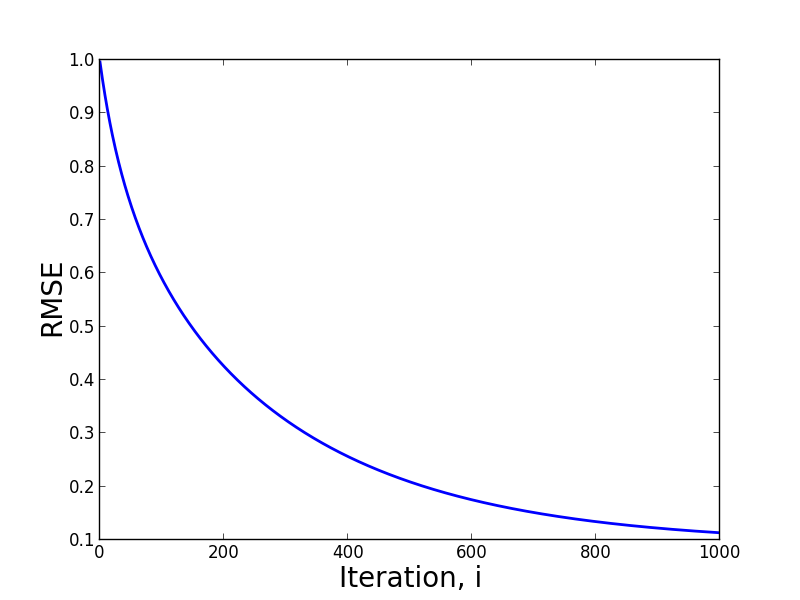# Collaborative Filtering With Uv Decomposition

Collaborative filtering is a technique for building recommender systems that relies only on a user's past behavior to make recommendations about what he or she should next in the future. There are two primary classes of solutions to the problem: nearest neighbor methods and latent factor models. Research has shown that latent factor models outperform nearest neighbor methods, so in this post, I'll work through implementing a latent factor model known as UV-factorization. UV-factorization is a type of matrix factorization, where a single large matrix is approximated by the product of two lower rank matrices.

## The problem

Mathematically, the problem begins with a large matrix, known as a utility matrix, $\boldsymbol{M}$, that contains behavioral data about individual users and the products or services they interact with. Each row in the matrix represents a user and each column represents a user's past interactions with specific products or services. Often times user interactions are characterized as ratings or purchases.

The solution to the problem is to determine which, out of all products each user has not interacted with, is the most likely to be preferred the next time the user chooses to perform an action, such as watching a movie or buying another pair of shoes. This is done by decomposing the utility matrix into two lower rank matrices, that when multiplied together, fill in the missing (user, item) pairs. $\boldsymbol{UV} = \boldsymbol{M}$

To estimate $\boldsymbol{U}$ and $\boldsymbol{V}$, we can define our objective function in terms of minimizing the squared error between each row of M and the inner product of the corresponding rows of $\boldsymbol{U}$ and $\boldsymbol{V}$. To prevent overfitting, we can also apply a regularization term. Mathematically, our objective function is defined as, $\sum_{(i,j)} (\boldsymbol{M}_{ij} - \boldsymbol{V}_j^T \boldsymbol{U}_i)^2 + \lambda( \|\boldsymbol{V}_j\|^2 + \|\boldsymbol{U}_i\|^2).$

To solve this objective, we can use stochastic gradient descent, originally presented here. After taking derivatives of the objective with respect to $\boldsymbol{U}$ and $\boldsymbol{V}$ we are left with the following pair of update rules: $\boldsymbol{U}_i \leftarrow \boldsymbol{U}_i + \alpha((\boldsymbol{M}_{ij} - \boldsymbol{V}_j^T \boldsymbol{U}_i)\boldsymbol{V}_j - \lambda \boldsymbol{U}_i) \\ \boldsymbol{V}_j \leftarrow \boldsymbol{V}_j + \alpha((\boldsymbol{M}_{ij} - \boldsymbol{U}_i^T \boldsymbol{V}_j)\boldsymbol{U}_i - \lambda \boldsymbol{V}_j),$ where $\alpha$ is the learning rate parameter and $\lambda$ is the regularization parameter. After estimating $\boldsymbol{U}$ and $\boldsymbol{V}$ by iterating over the known ($i,j$) pairs in the data, user $i$'s recommendation for product $j$ can be estimated by computing $\boldsymbol{U_i V_j^T}$.

## Implementation

As we've seen in other posts, implementing stochastic gradient descent is more or less trivial, once we have derived the update rules. The code below takes as input, a sparse scipy matrix and iterates over the data. The free parameters, f, lr and reg define the width of $\boldsymbol{U}$ and $\boldsymbol{V}$, the learning rate, $\alpha$, and the regularization parameter, $\lambda$, respectively.


def sgd_uv(util_mtx, f=5, lr=0.001, reg=0.1, max_iter=1000):
#get dimensions of util_mtx, which is a compressed sparse row matrix
r,c = util_mtx.shape
#initialize item matrix
v = np.random.rand(c,f)
#initialize user matrix
u = np.random.rand(r,f)
#fit the matrices with a fixed number of iterations
for c in xrange(max_iter):
for i in xrange(r):
for j in util_mtx[i].indices:
err = util_mtx[i,j] - np.dot(v[j], u[i])
v[j] = v[j] + lr*(err*p[i]-reg*v[j])
u[i] = u[i] + lr*(err*v[j]-reg*u[i])
return u,v



## Testing for convergence

To measure the algorithm's convergence, we can compute the root mean squared error (RMSE) after each iteration of SGD, shown below.


def rmse(util_mtx, u, v):
e = 0.
m = 0.
r,c = util_mtx.shape
for i in xrange(r):
for j in util_mtx[i].indices:
e += (util_mtx[i,j]-np.dot(v[j], u[i]))**2
m+=1
return np.sqrt(e/m)



In the code below, we create a sparse matrix, initialize it with random values, and then pass it to our learning algorithm.


#make a matrix
A = scipy.sparse.lil_matrix((100, 100))
#fill some of with random numbers
A[0, :10] = np.random.rand(10)
A[1, 10:20] = A[0, :10]
A.setdiag(np.random.rand(100))
#convert to compressed sparse row for quick row iterations
A = A.tocsr()
#fit the model
u,v,err_arr = sgd_uv(A)
#plot the error as a function of learning algorithm iteration
plt.plot(err_arr, linewidth=2)
plt.xlabel('Iteration, i', fontsize=20)
plt.ylabel('RMSE', fontsize=20)
plt.title('Components = '+str(j), fontsize=20)
plt.show()



The figure below plots the RMSE as a function of SGD iteration. The exponential decay early in the iteration history and leveling off towards the end suggests the algorithm is converging on a solution, which may be a local one.Note that to measure the performance of the algorithm, a fraction of the ($i,j$) pairs should be held out of the training process and be used as a test set, which I haven't done here.

## Conclusion

In this post I've focused on a relatively simple objective function, to simplify the presentation. In reality, a more complex objective might be required to achieve a desired level of performance. These objectives might include some implicit features, like age and gender, to work around the cold start problem.

machine learning

Archives